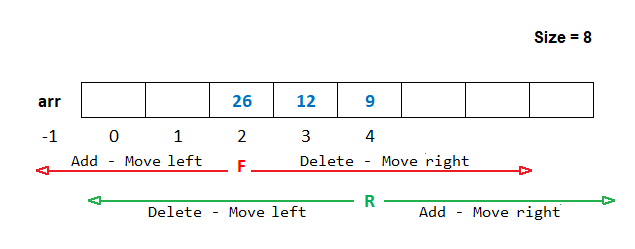# Double Ended Queue in C Programming

###### Data Structure in C

In this lesson, we will understand what is Double Ended Queue in C Programming and how to create them along with some examples.

## What is Double Ended Queue in C

A Double Ended Queue in C, also known as Deque, is a queue data structure in which insertion and deletion can be done from both left and right ends.From the above image of the deque, we can see that when we add an element from the rear end, the R moves towards the right and, when we delete an element from the rear end, the R moves towards the left.

Similarly, when we delete an element from the front end, the F moves towards the right and when we add an element from the front end, the F moves towards the left.## Operation on Circular Queue

There are four operations possible on the double ended queue.

• Add Rear When we add an element from the rear end.
• Delete Rear When we delete an element from the rear end.
• Add Front When we add an element from the front end.
• Delete Front When we delete an element from the front end.

Note: We will use the circular array to implement the Double Ended Queue.

### Add Rear Operation in Deque

For adding an element from the rear end first, we check if the value of te is equal to the size of the array then, we will display a message Queue is full, else we will increase the value of R by R=(R+1)%Size and add the element in the array at the new location of R and then increased the value of te by 1.

Here, te is a variable that keeps the total number of elements present in the array. When an element is added, the value of te is increased by 1. When an element has deleted, the value of te is decreased by 1.

#### Example

``````if(te==size)
{
printf("Queue is full\n");
}
else
{
R=(R+1)%size;
arr[R]=new_item;
te=te+1;
}``````

### Delete Rear Operation in Deque

For deleting an element from the rear end first, we check if the value of te is equal to 0 then, we will display a message Queue is empty, else we will check if the value of R is -1, then we will initialize the value of R=size-1. After that, we will display the deleted element on the screen and decrease the value of R and te by 1.

#### Example

``````if(te==0)
{
printf("Queue is empty\n");
}
else
{
if(R==-1)
{
R=size-1;
}
printf("Number Deleted From Rear End = %d",arr[R]);
R=R-1;
te=te-1;
}``````

### Add Front Operation in Deque

For adding an element from the front end first, we check if the value of te is equal to the size of the array then, we will display a message Queue is full, else we will check if the value of F is 0 then we will initialize the value of F=size-1, else decrease the value of F by 1. After that, add the element in the array at the new location of F and then increased the value of te by 1.

#### Example

``````if(te==size)
{
printf("Queue is full");
}
else
{
if(F==0)
{
F=size-1;
}
else
{
F=F-1;
}
arr[F]=new_item;
te=te+1;
}``````

### Delete Front Operation in Deque

For deleting an element from the front end first, we check if the value of te is equal to 0 then, we will display a message Queue is empty, else we will display the deleted element on the screen and then we will increase the value of F by F=(F+1)%Size and then decrease the value of te by 1.

#### Example

``````if(te==0)
{
printf("Queue is empty");
}
else
{
printf("Number Deleted From Front End = %d",arr[F]);
F=(F+1)%size;
te=te-1;
}``````

## Program of Deque using Circular Array

Below is the complete program of double ended queue in C using a circular array having size 5.

#### Deque Program in C using Circular Array

``````#include <stdio.h>
#include <conio.h>
#include <stdlib.h>
#define size 5

int main()
{
int arr[size],R=-1,F=0,te=0,ch,n,i,x;

for(;;)		// An infinite loop
{
system("cls");		// for clearing the screen
printf("F=%d  R=%d\n\n",F,R);
printf("2. Delete Rear\n");
printf("4. Delete Front\n");
printf("5. Display\n");
printf("6. Exit\n");
printf("Enter Choice: ");
scanf("%d",&ch);

switch(ch)
{
case 1:
if(te==size)
{
printf("Queue is full");
getch();	// pause the loop to see the message
}
else
{
printf("Enter a number ");
scanf("%d",&n);
R=(R+1)%size;
arr[R]=n;
te=te+1;
}
break;

case 2:
if(te==0)
{
printf("Queue is empty");
getch();	// pause the loop to see the message
}
else
{
if(R==-1)
{
R=size-1;
}
printf("Number Deleted From Rear End = %d",arr[R]);
R=R-1;
te=te-1;
getch();	// pause the loop to see the number
}
break;

case 3:
if(te==size)
{
printf("Queue is full");
getch();	// pause the loop to see the message
}
else
{
printf("Enter a number ");
scanf("%d",&n);
if(F==0)
{
F=size-1;
}
else
{
F=F-1;
}
arr[F]=n;
te=te+1;
}
break;

case 4:
if(te==0)
{
printf("Queue is empty");
getch();	// pause the loop to see the message
}
else
{
printf("Number Deleted From Front End = %d",arr[F]);
F=(F+1)%size;
te=te-1;
getch();	// pause the loop to see the number
}
break;

case 5:
if(te==0)
{
printf("Queue is empty");
getch();	// pause the loop to see the message
}
else
{
x=F;
for(i=1; i<=te; i++)
{
printf("%d ",arr[x]);
x=(x+1)%size;
}
getch();	// pause the loop to see the numbers
}
break;

case 6:
exit(0);
break;

default:
printf("Wrong Choice");
getch();	// pause the loop to see the message
}
}
return 0;
}``````

In the above program, we have defined a macro named size having value 5 using the statement #define. We can use the word size to declare the size of the array as int arr[size]. When we run the above program, the word size will be replaced by its value 5. So the size of the array will be 5.